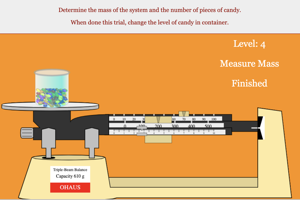Information About The Program
Candy Container Lab (Lab)This lab is used as an introductory lab for students learning how to create and use equations based on experimental data. Students will collect data on the number of pieces of candy in a container and the corresponding mass of the system. Students will then graph that data to find the mass of the container and the average mass of 10 pieces of candy. Finally, students will use the equation to make predictions for situations that were not collected directly.
Below are any Resources that go with this program